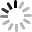# Google 試算表 (6) - 網頁寫入資料

## parameter 資料格式

``````var data = [
['123', '456', '789'],
['x', 'y', 'z']
];
var parameter = {};
parameter.data = data.toString();
``````

``````function doGet(e) {
var params = e.parameter;
var url = params.url;
var name = params.name;
var d = params.data;   // 原始 data，字串格式
var row = params.row;  // 由原始 data 判斷需要插入幾列
var column = params.column; // 由原始 data 判斷每一列有幾欄
var insertType = params.insertType;  // 插入在上方，或插入在下方
var lastRow = SheetName.getLastRow();  // 讀取最後一列的列數
var range, data, arr;
}
``````

``````function doGet(e) {
var params = e.parameter;
var url = params.url;
var name = params.name;
var d = params.data;   // 原始 data，字串格式
var row = params.row;  // 由原始 data 判斷需要插入幾列
var column = params.column; // 由原始 data 判斷每一列有幾欄
var insertType = params.insertType;  // 插入在上方，或插入在下方
var lastRow = SheetName.getLastRow();  // 讀取最後一列的列數
var range, data, arr;

if(d.indexOf(',')!=-1){
arr = d.split(','); // 把原始資料用 , 分割成陣列
data=[];
for(var i=0; i<row; i++){
data[i]=[];
for(var j=0; j<column; j++){
data[i].push(arr[i*column+j]); // 藉由 row 和 column 變成二維陣列
}
}
}else{
data = [[d]];
}
}
``````

``````function doGet(e) {
var params = e.parameter;
var url = params.url;
var name = params.name;
var d = params.data;   // 原始 data，字串格式
var row = params.row;  // 由原始 data 判斷需要插入幾列
var column = params.column; // 由原始 data 判斷每一列有幾欄
var insertType = params.insertType;  // 插入在上方，或插入在下方
var lastRow = SheetName.getLastRow();  // 讀取最後一列的列數
var range, data, arr;

if(d.indexOf(',')!=-1){
arr = d.split(','); // 把原始資料用 , 分割成陣列
data=[];
for(var i=0; i<row; i++){
data[i]=[];
for(var j=0; j<column; j++){
data[i].push(arr[i*column+j]); // 藉由 row 和 column 變成二維陣列
}
}
}else{
data = [[d]];
}

if(insertType=='top'){
SheetName.insertRowsBefore(1,row);
range = SheetName.getRange(1,1,row,column);
}else if(insertType=='bottom'){
range = SheetName.getRange(lastRow+1,1,row,column);
}

range.setValues(data);

return ContentService.createTextOutput(true);
}
``````

``````function myFunction() {
var data = [['123','456','789']];
doGet({
parameter:{
url: '試算表網址',
name: '工作表名稱',
data: data.toString(),
insertType: 'bottom',
row:data.length,
column:data.length
}
});
}
``````## 網頁寫入資料``````Google App Script：<input id="appUrl"><br/>

<select id="type">
<option value="top">從上寫入</option>
<option value="bottom">寫入在最後一列</option>
</select>
<br/>

<table>
<tr>
<td>123</td>
<td>456</td>
<td>789</td>
</tr>
</table>
<button id="sendBtn">寫入</button>
``````

JavaScript 和剛剛的 debug.gs 大同小異，主要就是把欄位的資料帶入 parameter，最後用`\$.get`的方法送出，完成後，填入對應的網址，點選送出，應該就可以看到資料出現在試算表內了。

``````var appUrl = \$('#appUrl'),
sheetsUrl = \$('#sheetsUrl'),
sheetName = \$('#sheetName'),
type = \$('#type'),
sendBtn = \$('#sendBtn'),
show = \$('#show');
var data = [['123','456','789']];
var parameter = {};
sendBtn.on('click', function() {
parameter = {
url: sheetsUrl.val(),
name: sheetName.val(),
data: data.toString(),
row: data.length,
column: data.length,
insertType: type.val()
};
\$.get(appUrl.val(), parameter);
});
``````